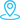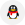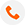您的位置：新闻资讯 >文章内容### 1. 忘记写冒号

``if spam == 42    print('Hello!')``

### 2. 误用 “=” 做等值比较

“=”是给变量赋值，“==”才是判断两个值是否相等：

``score = 60if score = 60:    print ('passed')``

``score = 60if score == 60:    print ('passed')``

### 3. 变量没有定义：

``if age >= 18:    print ('adult')print ('END')``

``age = 20if age >= 18:    print ('adult')print ('END')``

### 4. 字符串与非字符串连接

``num = 12print('I have ' + num+ ' apples.')``

``num = 12print('I have ' + str(num)+ ' apples.')``

### 5. 列表的索引位置

``spam = ['cat', 'dog', 'mouse']print(spam)``

``spam = ['cat', 'dog', 'mouse']print(spam)``

### 6. 使用自增 “++” 自减 “—”

``spam = 0spam++``

``spam = 0spam += 1``

### 7. 使用关键字命名变量

Python 3中一共33个关键字：

False，None，True，and，as，assert，break，class，continue，def，del，elif，else，except，finally，for，from，global，if，import，in，is，lambda，nonlocal，not，or，pass，raise，return，try，while，with，yield

### 8. 索引元素位置时忘记调用 len 方法

``fam = ['dad', 'mom', 'son']for i in range(fam):print(fam[i])``

``fam = ['dad', 'mom', 'son']for i in range(len(fam)):print(fam[i])``

### 9. 函数中的局部变量赋值前被使用

``num = 42def myFunction():    print(num)    num = 100myFunction()``

### 10. 缩进问题

（1）

``print('Hello')    print('World!')``

``print('Hello')print('World!')``

（2）

``if spam == 42:    print('Hello')  print('World!')``

``if spam == 42:    print('Hello')    print('World!')``

``if spam == 42:    print('Hello')print('World!')``

（3）在Python 3中，缩进的时候，不能Tab和空格混用，每个缩进层次应该选择只使用Tab或者只使用空格。182938038113316264505